# Ditkin set

A closed subsetof a locally compact spaceis called a Ditkin set (with respect to a regular function algebra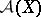defined on; cf. Algebra of functions) if eachvanishing oncan be approximated, arbitrarily closely, by functions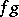with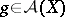andvanishing "near"(i.e. on a neighbourhood of). The notion of a Ditkin set is closely related to, but more restrictive than, that of a set of spectral synthesis (cf. Spectral synthesis): for such a set the requirement is that each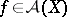vanishing oncan be approximated by functionsvanishing near.

The closed ideal of allvanishing onis usually denoted by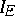. Denoting the ideal of allvanishing nearby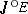and its closure by, one has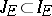. Nowis a set of spectral synthesis if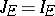, whereasis a Ditkin set if each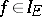belongs to the closure of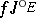(or, equivalently, to the closure of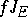). It is a famous open problem (as of 2000) whether (in specific cases) each set of spectral synthesis is actually a Ditkin set (this problem may be called the synthesis-Ditkin problem; in [a1] it is called the-set--set problem).

Ditkin sets were first studied for the Fourier algebra, with the norm defined by; here,is any locally compact Abelian group,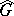is its dual group, and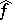is the Fourier transform of(cf. also Harmonic analysis; Fourier transform). A.P. Calderón (1956) studied this kind of set in an effort to obtain results about sets of spectral synthesis. Therefore, Ditkin sets are sometimes called Calderón sets or-sets; cf. [a4] and [a10], respectively. The name "Ditkin set" , attributed in [a6], p. 183, to C.S. Herz, refers to work of V.A. Ditkin (1910–1987) in his seminal paper [a2]; results from this paper were later studied and generalized in [a11]. In [a9] the term Wiener–Ditkin set is used.

The union of two Ditkin sets is again a Ditkin set; this follows easily from a triangle inequality like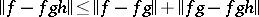. More generally, if a closed set is the union of countably many Ditkin sets, then it is again a Ditkin set. In contrast, for most function algebras it is unknown (as of 2000) whether the union of two sets of spectral synthesis is again of spectral synthesis: this is the famous union problem for this class of sets. Of course, the union problem becomes trivial if the synthesis-Ditkin problem gets a positive answer. Also, ifand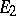are sets of spectral synthesis such that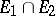is a Ditkin set, then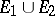is a set of spectral synthesis.

It is also easy to prove that if the boundary of a closed setis a Ditkin set, then so isitself; cf. [a10] (for the case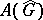), [a9] or [a8].

Ditkin sets are of particular interest ifsatisfies Ditkin's condition, i.e. if single points are Ditkin sets for. This notion is older than that of a Ditkin set; cf., e.g., [a7], p. 86. Ifhas approximate units (i.e. if eachcan be approximated by functionswith), thensatisfies Ditkin's condition if and only if for eachand eachsuch that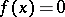(i.e. such thatbelongs to the maximal ideal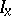), the zero function can be approximated, arbitrarily closely, by functions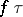with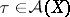andequalsnear. It follows that ifsatisfies Ditkin's condition, then closed scattered sets (cf. Scattered space) are Ditkin sets.

The following results can be found in [a8], Sec. 7.4. First, closed subgroups ofare Ditkin sets for the Fourier algebra, and the same result still holds for certain Beurling algebras. Secondly, the following injection theorem for Ditkin sets holds: Ifis a closed subgroup of, andis a closed subset of, thenis a Ditkin set forif and only if it is one for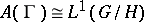, where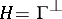, the subgroup oforthogonal to(cf. also Orthogonality).

In the literature a more restrictive class of sets is also considered, especially in the case of the Fourier algebra. A closed setis called a strong Ditkin set if there exists a net(cf. also Net (directed set)), bounded in the operator norm (i.e. the mappingis bounded), such that. Ifis metrizable (cf. Metrizable space), then one can require, equivalently, the existence of a sequence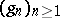insuch that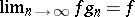for all, the boundedness in operator norm then being automatically satisfied, by the uniform boundedness theorem (cf. Uniform boundedness)

Strong Ditkin sets were first considered by I. Wik [a12]. Subsequently it was proved that a closed subsetofwithout interior is a strong Ditkin set forif and only if E belongs to the coset ring of(cf., e.g., [a4], [a3], [a8] for details).

A closed interval in the circle group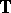is a strong Ditkin set; cf. [a12]. Therefore, it is essential, for the criterion above, to consider closed sets with empty interior. Also, a line segment in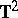is not a strong Ditkin set for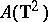, because it has empty interior but does not belong to the coset ring. Consequently, the above-mentioned injection theorem does not hold for strong Ditkin sets.

Ifis not a set of spectral synthesis, then only functions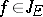have a chance of being approximable in the Ditkin sense. This motivates the following definition, given in [a8]. A closed setis called a Ditkin set in the wide sense if eachcan be approximated by functionswith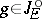. This notion is, in a way, more natural than that of a Ditkin set; but in 1956 it was not yet known that sets not of spectral synthesis abound in the case of the Fourier algebra: Malliavin's result (cf. Spectral synthesis) dates from 1959. It is not known in general (for instance in the case of the Fourier algebra) whether all closed subsets are Ditkin sets in the wide sense. This problem is a natural generalization of the synthesis-Ditkin problem.